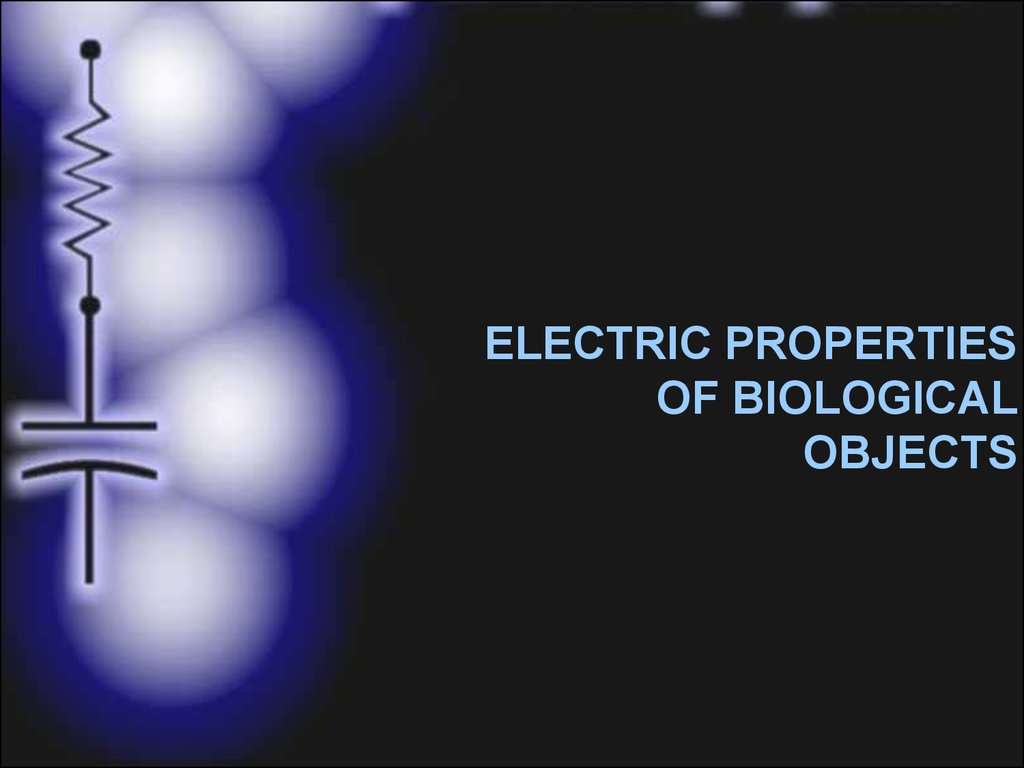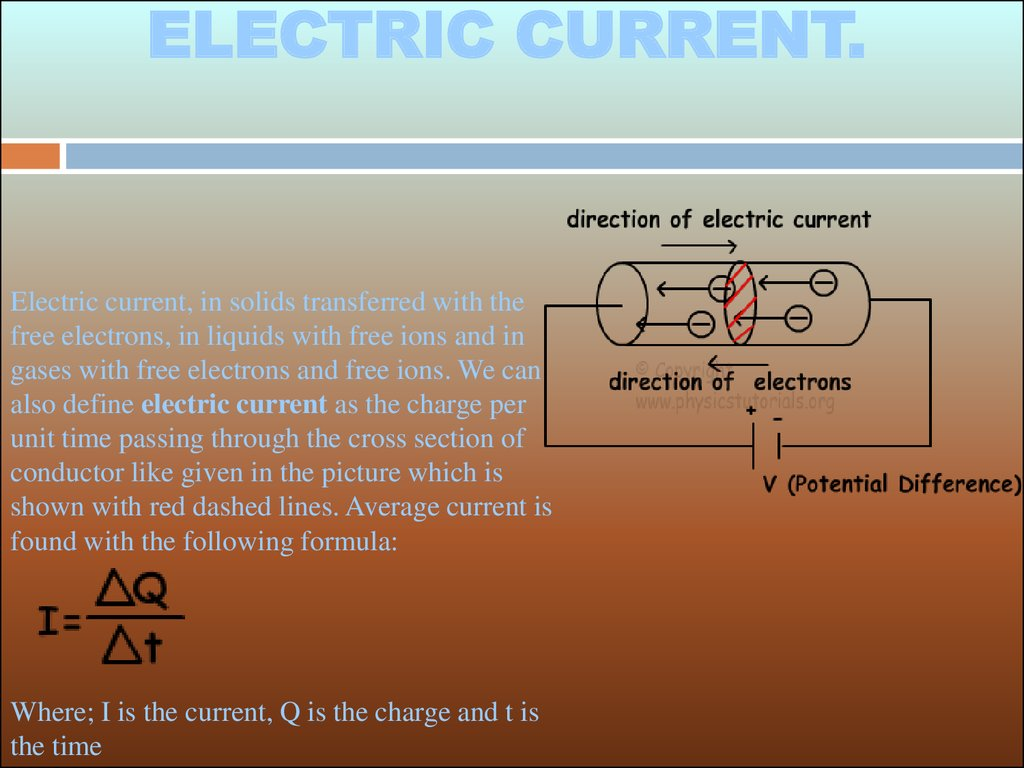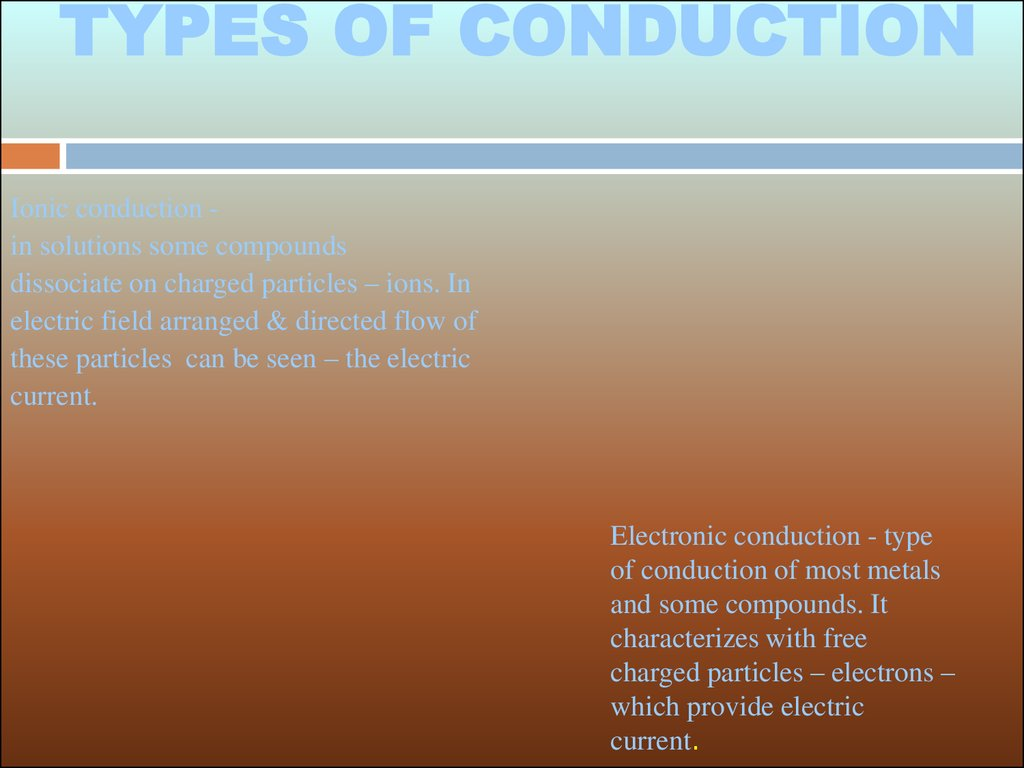# Electric properties of biological objects

## 1.

ELECTRIC PROPERTIES
OF BIOLOGICAL
OBJECTS

## 2.

ELECTRIC CURRENT.
Electric current, in solids transferred with the
free electrons, in liquids with free ions and in
gases with free electrons and free ions. We can
also define electric current as the charge per
unit time passing through the cross section of
conductor like given in the picture which is
shown with red dashed lines. Average current is
found with the following formula:
Where; I is the current, Q is the charge and t is
the time

## 3.

TYPES OF CONDUCTION
Ionic conduction in solutions some compounds
dissociate on charged particles – ions. In
electric field arranged & directed flow of
these particles can be seen – the electric
current.
Electronic conduction - type
of conduction of most metals
and some compounds. It
characterizes with free
charged particles – electrons –
which provide electric
current.

## 4.

RESISTANCE
OHM’s LAW
I = U/R
ACTIVE
RESISTANCE
R = rl/S
RESISTANCE - defines counteraction to
charges’ flow. It depends on length , cross
section and material of conductor .

## 5.

+q
-q
CAPACITY – defines potentials difference between two
isolated bodies if they have charges of the same value but
opposite signs. Capacity of Capacitor depends on area of
plates (S), distance between them(d) & dielectric
permeability of isolator material(e).
U = q/C
C = eS/d
Alternating current resistance Capacity resistance
X = 1/wC
L
C

## 6.

Alternating current
resistance Inductive resistance X = wL
INDUCTIVITY – characterizes electromotive force which opposes
current change in the electric circuit. Inductivity of inductivity coil
depends on coil length (l), quantity of coil turns (N), turns’ area &
magnetic permeability of the core(m).
U = - L di/dt
L = mN S/l

## 7.

DIELECTRICS POLARIZATION
Cells & tissues
polarization.

## 8.

IMPEDANCE PLETISMOGRAPHY
IMPEDANCE PLETISMOGRAPHY (Rheography) - noninvasive method of
organs’ blood supply examination. The greater the tissue blood income the
smaller the resistance in this tissue. For pletismography registration the
alternating electric current with frequency 50-100кHz & with intensity less
than 10 мкА is used. It is generated by special generator.

## 9.

RHEOGRAM REGISTRATION METHODS
ELECTRIC CURRENT
ELECTRODES
MEASUREMENT
ELECTRODES
Tetrapolar method
Scheme
Of bridge
measurement

## 10.

RHEOGRAM ANALYSIS
anacrotic wave
Catacrotic wave
incision
There are two parts on rheogram: sistolic
(anacrotic wave) & diastolic (catacrotic
wave). First part (anacrotic wave) is caused
by inflow of blood to the organ. Second
part of rheogram (anacrotic wave) is caused
by outflow of the blood from the organ.
The curve on the declining part of the
graph is called incision. To analyze the
rheogram its amplitudes and time periods
should be measured. These characteristics
shows the tonus state of vessels, their
elasticity, systolic volume. Besides the
special rheographic parameters are
measured.